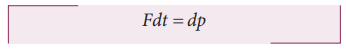Home | | Physics 11th std | Impulsive force or Impulse

# Impulsive force or Impulse

Physics : Laws of Motion : Impulsive force or Impulse

Impulse

If a very large force acts on an object for a very short duration, then the force is called impulsive force or impulse.

If a force (F) acts on the object in a very short interval of time (t), from Newton’s second law in magnitude formIntegrating over time from an initial time ti to a final time t f , we getIf the force is constant over the time interval, thenEquation (3.24) is called the ‘impulse-momentum equation’.

For a constant force, the impulse is denoted as J = F∆ t and it is also equal to change in momentum ( ∆p) of the object over the time interval ∆t.The average force acted on the object over the short interval of time is defined byFrom equation (3.25), the average force that act on the object is greater if ∆t is smaller. Whenever the momentum of the body changes very quickly, the average force becomes larger.

The impulse can also be written in terms of the average force. Since ∆p is change in momentum of the object and is equal to impulse (J), we haveThe graphical representation of constant force impulse and variable force impulse is given in Figure 3.21.Illustration

1. When a cricket player catches the ball, he pulls his hands gradually in the direction of the ball’s motion. Why?

If he stops his hands soon after catching the ball, the ball comes to rest very quickly. It means that the momentum of the ball is brought to rest very quickly. So the average force acting on the body will be very large. Due to this large average force, the hands will get hurt. To avoid getting hurt, the player brings the ball to rest slowly.2. When a car meets with an accident, its momentum reduces drastically in a very short time. This is very dangerous for the passengers inside the car since they will experience a large force. To prevent this fatal shock, cars are designed with air bags in such a way that when the car meets with an accident, the momentum of the passengers will reduce slowly so that the average force acting on them will be smaller.3. The shock absorbers in two wheelers play the same role as airbags in the car. When there is a bump on the road, a sudden force is transferred to the vehicle. The shock absorber prolongs the period of transfer of force on to the body of the rider. Vehicles without shock absorbers will harm the body due to this reason.

4. Jumping on a concrete cemented floor is more dangerous than jumping on the sand. Sand brings the body to rest slowly than the concrete floor, so that the average force experienced by the body will be lesser.### Example 3.16

An object of mass 10 kg moving with a speed of 15 m s−1 hits the wall and comes to rest within

a. 0.03 second

b. 10 second

Calculate the impulse and average force acting on the object in both the cases.

### SolutionWe see that, impulse is the same in both cases, but the average force is different.

Study Material, Lecturing Notes, Assignment, Reference, Wiki description explanation, brief detail
11th Physics : UNIT 3 : Laws of Motion : Impulsive force or Impulse |